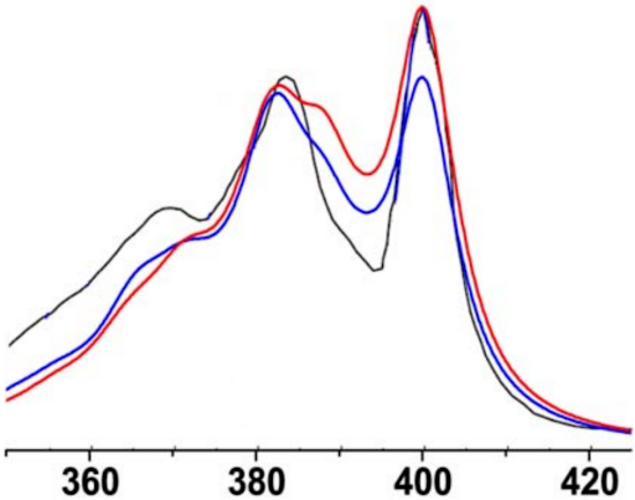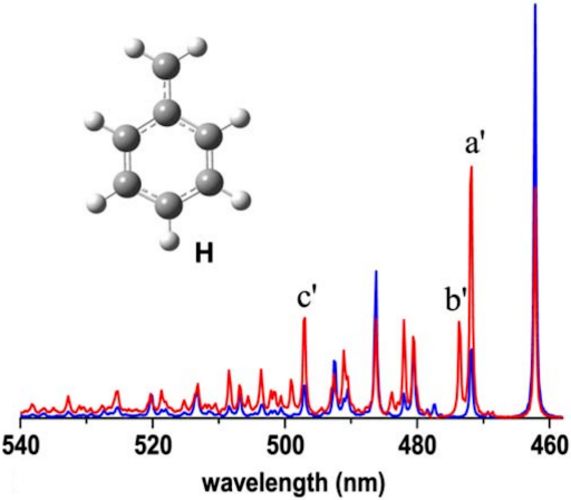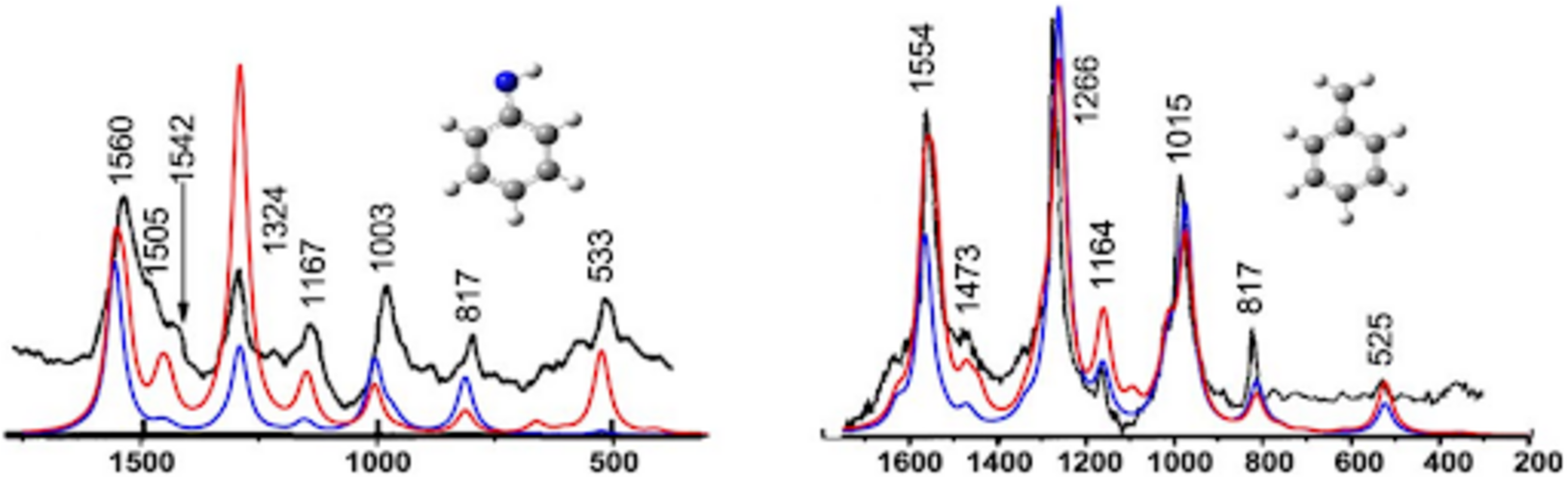# Simulating Vibrationally Resolved Electronic and Raman Spectra

A built-in module for computing vibrational progressions affords calculations of vibrationally resolved one-photon absorption (OPA), one-photon emission (OPE), and resonance Raman spectra (RRS):

• Effective algorithm reduces computational costs by recasting sum-over-state expressions into the time-domain, thus avoiding explicit calculation of individual excited states.

• Different levels of theoretical treatment are implemented:

• Franck-Condon (FC): includes only the zero-order term of the transition dipole moment.

• Franck-Condon-Herzberg-Teller (FCHT): includes the zero- and first-order terms of the transition dipole moment.

• Vertical gradient (VG): the excited state potential energy surface is approximated by a shift to the ground-state surface.

• Duschinsky rotation: includes mode mixing effect.Calculated FC (blue) and FCHT (red) absorption spectra of D$$_0\rightarrow$$D$$_3$$ transition for phenoxyl radicalCalculated FC (blue) and FCHT (red) emission spectra of D$$_1\rightarrow$$D$$_0$$ transition for benzyl radical